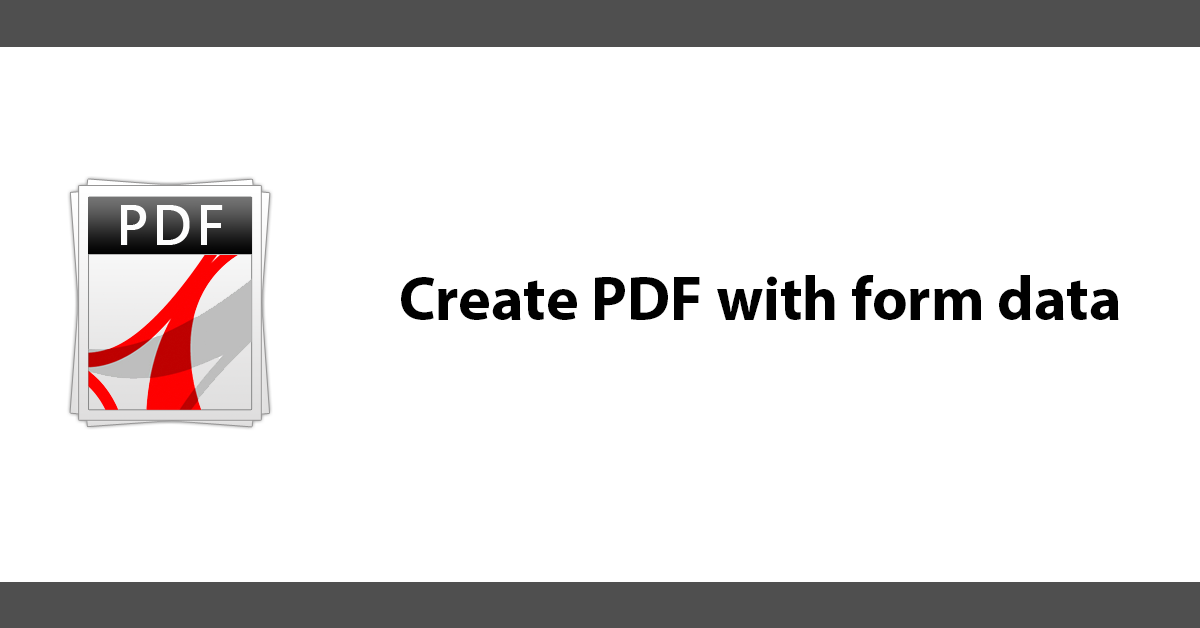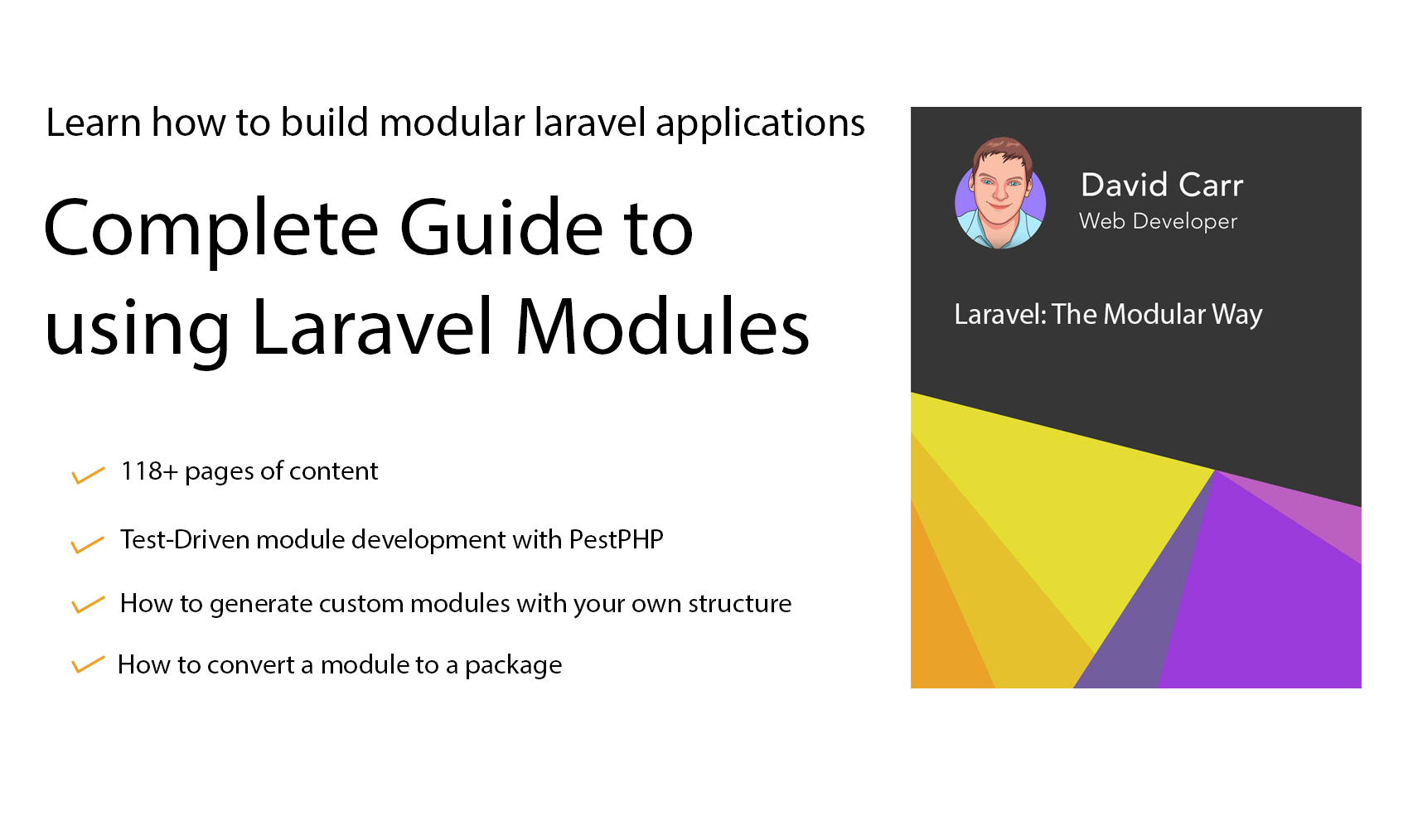# Create PDF with form data

It’s possible to populate a PDF using information provided by a form using the MPDF library, this tutorial will show you how.

First here is a simple form with a couple of fields.

``````<form action='' method='post'>
<p><label>Name</label><br><input type='text' name='name' value=''></p>
<p><label>Email</label><br><input type='text' name='email' value=''></p>
<p><input type='submit' name='submit' value='Submit'></p>
</form>``````

Now we have a form with a name and email address we can proccess it. Check if the form has been submitted, collect the form data, make sure it passes the validation:

``````<?php
if(isset(\$_POST['submit'])){

//collect form data
\$name = \$_POST['name'];
\$email = \$_POST['email'];

//check name is set
if(\$name ==''){
\$error[] = 'Name is required';
}

//check for a valid email address
if(!filter_var(\$email, FILTER_VALIDATE_EMAIL)){
}``````

If the checks all pass.

``````//if no errors carry on
if(!isset(\$error)){``````

Next make the HTML data for the PDF, I’ve chosen to use ob_start add ob_get_clean which are build in PHP functions that start a buffer and then collect the buffer contents and returns them. This is very handy as it allows for html to be written then stored rather then displayed.

``````//create html of the data
ob_start();
?>

<h1>Data from form</h1>
<p>Name: <?php echo \$name;?></p>
<p>Email: <?php echo \$email;?></p>

<?php
\$body = ob_get_clean();``````

Now create the PDF, set the PDF to ignore any invalid UTF-8 data.
Next include the MDPF library.
Create a new instance.
Write the html to the PDF
Finally output the PDF, here we have a few options:

Save the PDF on the server by giving a filename and a flag or F:

``\$mpdf->Output("mydata.pdf",'F');``

``\$mpdf->Output('demo.pdf','D');``

Lastly to output the PDF to the browser:

``\$mpdf->Output();``

Here we create the PDF and offer the PDF as a download.

``````\$body = iconv("UTF-8","UTF-8//IGNORE",\$body);

include("mpdf/mpdf.php");
\$mpdf=new \mPDF('c','A4','','' , 0, 0, 0, 0, 0, 0);

//write html to PDF
\$mpdf->WriteHTML(\$body);

//output pdf
\$mpdf->Output('demo.pdf','D');``````

If the validation was not passed loop through the error array and show the errors.

``````//if their are errors display them
if(isset(\$error)){
foreach(\$error as \$error){
echo "<p style='color:#ff0000'>\$error</p>";
}
}``````

Here is the full script:

``````<?php
if(isset(\$_POST['submit'])){

//collect form data
\$name = \$_POST['name'];
\$email = \$_POST['email'];

//check name is set
if(\$name ==''){
\$error[] = 'Name is required';
}

//check for a valid email address
if(!filter_var(\$email, FILTER_VALIDATE_EMAIL)){
}

//if no errors carry on
if(!isset(\$error)){

//create html of the data
ob_start();
?>

<h1>Data from form</h1>
<p>Name: <?php echo \$name;?></p>
<p>Email: <?php echo \$email;?></p>

<?php
\$body = ob_get_clean();

\$body = iconv("UTF-8","UTF-8//IGNORE",\$body);

include("mpdf/mpdf.php");
\$mpdf=new \mPDF('c','A4','','' , 0, 0, 0, 0, 0, 0);

//write html to PDF
\$mpdf->WriteHTML(\$body);

//output pdf
\$mpdf->Output('demo.pdf','D');

//open in browser
//\$mpdf->Output();

//save to server
//\$mpdf->Output("mydata.pdf",'F');

}
}

//if their are errors display them
if(isset(\$error)){
foreach(\$error as \$error){
echo "<p style='color:#ff0000'>\$error</p>";
}
}
?>

<form action='' method='post'>
<p><label>Name</label><br><input type='text' name='name' value=''></p>
<p><label>Email</label><br><input type='text' name='email' value=''></p>
<p><input type='submit' name='submit' value='Submit'></p>
</form>``````Help support the blog so that I can continue creating new content!